# Formative Assessment- Rational Numbers Class 8 Notes | EduRev

## Class 8 : Formative Assessment- Rational Numbers Class 8 Notes | EduRev

The document Formative Assessment- Rational Numbers Class 8 Notes | EduRev is a part of the Class 8 Course Class 8 Mathematics by VP Classes.
All you need of Class 8 at this link: Class 8

Ques 1: Match the following:

 Column A Column B (a) The rational number having no reciprocal is: (i) 1 (b) The product of a rational number (other than zero) and its reciprocal is: (ii) 2 (c) The reciprocal of (–1) is: (iii) 0 (d) The product of (–3) and the negative reciprocal of 1(½) is: (iv) – 1 (e) On a number line the rational number equidistant from 0 and –1 is: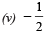Ques 2: Complete the following crossword puzzle using the given directions.

Across:
(1) The negative of a rational number is called its ___________.
(2) A number of the form p/q, where p and q are integers and q ≠ 0, is called a ______.

Down: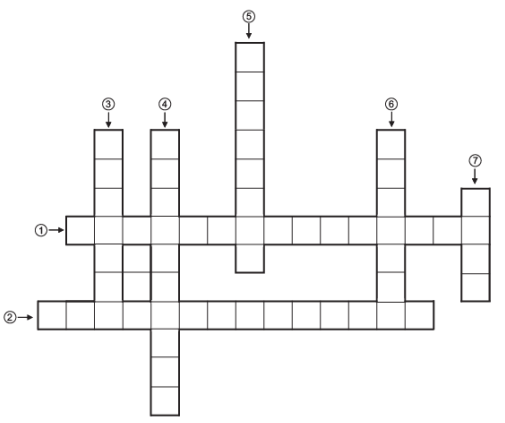(3) The __________ of a rational number and its reciprocal is 1.

(4) The multiplicative inverse of a number is also called its__________.

(5) Zero is also called the __________identity for rational numbers.

(6) If the product of two rational numbers is 1, then they are called multiplicative __________of each other.

(7) The rational number __________is the additive identity for rational numbers.

Ques 3: Choose the correct answer for each of the following:

(i) The sum of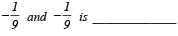(a) 0
(b) 1
(c) 2/9
(d) -2/9

(ii) The product of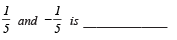(a) 0
(b) 1
(c) 1/25
(d) -1/25

(iii) The multiplicative inverse of 1/6 is _____________
(a) – 6
(b) 6
(c)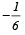(d) 1
(iv) The rational number equivalent to 9/-81 is :

(a) 18/162
(b) -81/162
(c)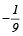(d) 1/9
(v) 0 reduced by 1/2 is
(a) 1/2
(b)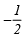(c) 2
(d) – 2

Ques 4: (i) Which of 0 or 1 is the multiplicative identity for rational numbers?
(ii) Which of 0 or 1 is the additive-identity for rational numbers?
(iii) Is 0 a rational number?
(iv) How many rational numbers can exist between 0 and –1?
(v) Is a rational number the quotient of two integers such that the denominator is a non-zero integer?
(vi) Is there any rational number which when multiplied by 0 gives 1?
(vii) Which rational numbers are their own reciprocals?
(viii) Do ‘a - 0’ and  ‘a - 0’ represent the same rational number?
(ix) Is there a rational number that is equal to its negative? If yes, write it.
(x) What is the reciprocal of the reciprocal of 1/2 ?

ANSWERS:

1. (a) → (iii)
(b) → (i)
(c) → (iv)
(d) → (ii)
(e) → (v)

2. (1) → ADDITIVE-INVERSE
(2) → RATIONAL-NUMBER
(3)→PRODUCT
(4) → RECIPROCAL
(5) → ADDITIVE
(6) → INVERSE
(7) → ZERO

3. (i) (d)
(ii) (d)
(iii) (b)
(iv) (c)
(v) (b)

4. (i) 1
(ii) 0
(iii) Yes
(iv) Unlimited
(v) Yes
(vi) No
(vii) 1 and –1
(viii) No

(ix) Yes, 0
(x) 1/2

Offer running on EduRev: Apply code STAYHOME200 to get INR 200 off on our premium plan EduRev Infinity!

93 docs|16 tests

,

,

,

,

,

,

,

,

,

,

,

,

,

,

,

,

,

,

,

,

,

;